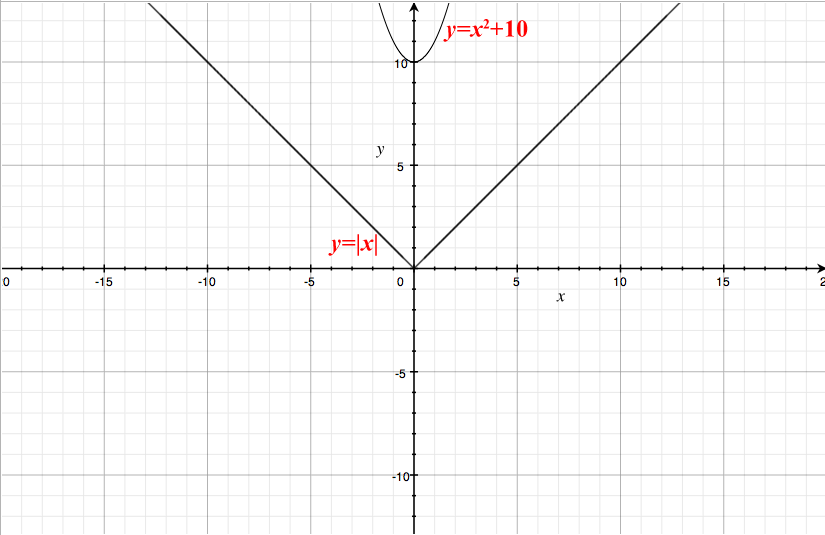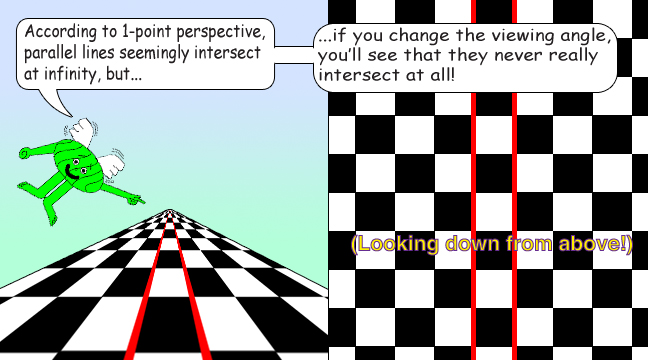# Impossible Equations

## There are functions that never intersect because they're parallel to each other. (Especially if they're linear functions, but some non-linear functions never intersect either.)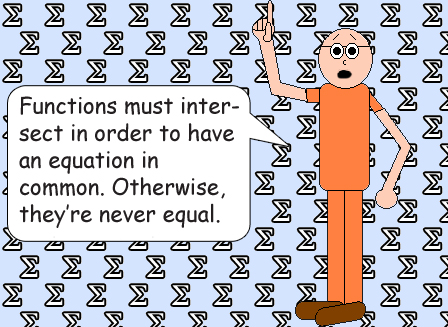## Below are some examples of non-intersecting function pairs:x is never equal to x + 1 because these 2 linear functions are parallel to each other.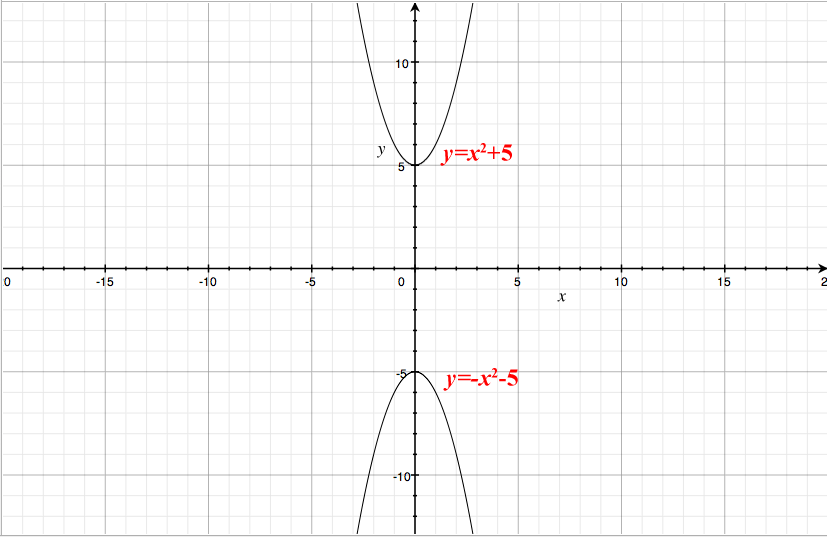x2 + 5 is never equal to -x2 - 5 because these 2 quadratic functions don't intersect.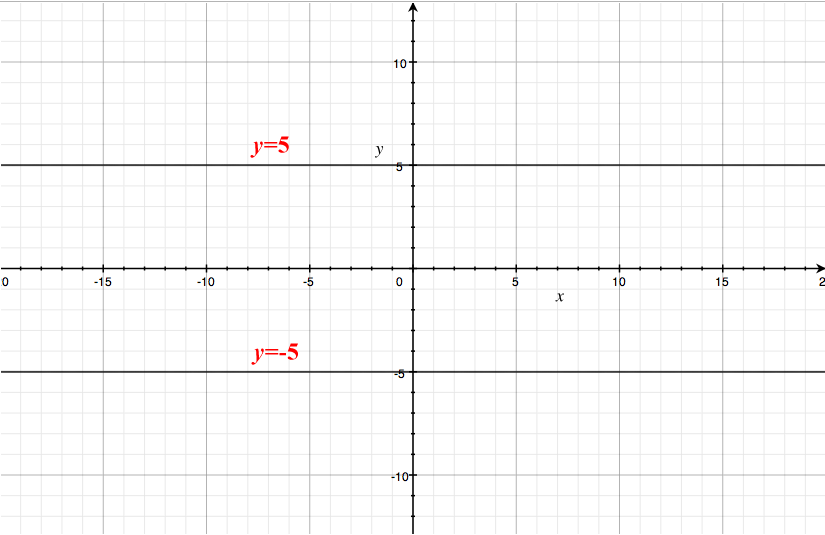These 2 functions are constant functions. Obviously, 5 ≠ -5 because they're 2 different numbers. (But they are additive inverses.)Here's a pair of vertical x-lines. This is more proof that 2 different numbers are never equal.